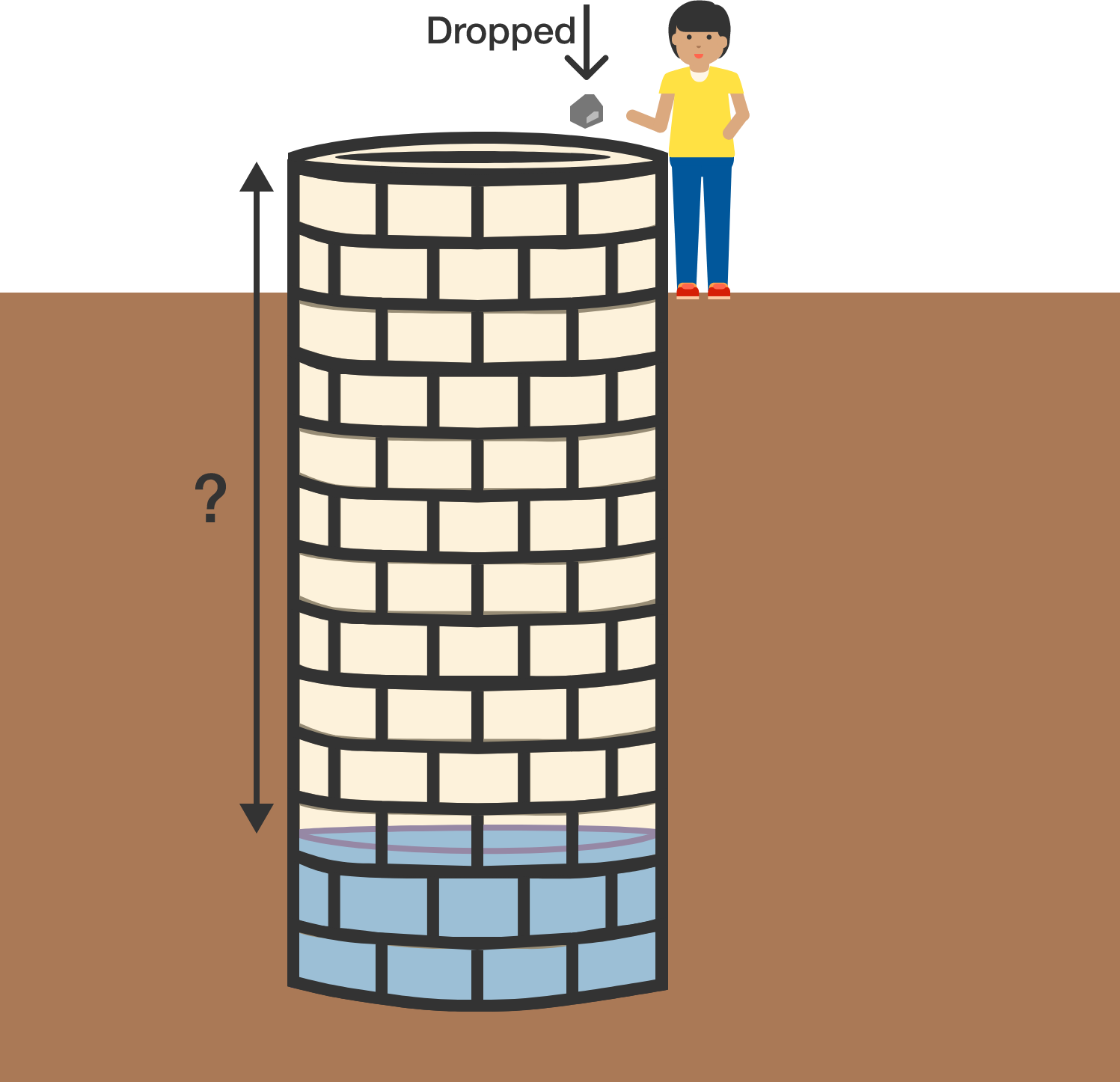# A classical mechanics problem by Nazmus sakibSelah dropped a stone into a deep well. After 3 seconds, she heard it hit the water.

If the speed of sound is $\SI[per-mode=symbol]{300}{\meter\per\second}$, determine the depth to the surface of the water. Give your answer in meters to 1 decimal place.

Take $g = \SI[per-mode=symbol]{9.81}{\meter\per\second\squared}.$

×